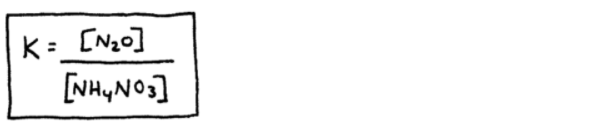# S13E3 - Equilibrium Expressions for Heterogeneous Equilibrium

Thus far we've only been discussing equilibrium situations in which all reactants and products have been in the gas phase.

These have all been instances of homogeneous equilibria, where only one phase is involved.

## Heterogeneous Equilibrium

Many equilibria however, involve more than one phase.  Here's an example of a heterogeneous equilibrium...

ex:    CaCO3(s)  ⇌  CaO(s)  +  CO2(g)The correct equilibrium expression is K = [CO2]

Why?

Because the concentrations of pure substances such as liquids and solids cannot change!

The take-home message here, is the following:

"Equilibrium expressions only include aqueous (aq) and gaseous (g) reactants and products."

-----

## Heterogeneous Equilibrium Examples

ex:  Write equilibrium expressions for Kc and Kp

( K is the same thing as Kc )

a.   P4(s)  +  5O2(g)  ⇌  P4O10(s)-----

b.   NH4NO3(s)  ⇌  N2O(g)  +  2H2O(g)-----

c.    NH4NO3(aq)  ⇌  N2O(g)  +  2H2O(l)=====

Coming up next as we continue with SECTION 13 - Chemical Equilibrium,

We'll talk about the Extent of a Reaction, and the Reaction Quotient, Q